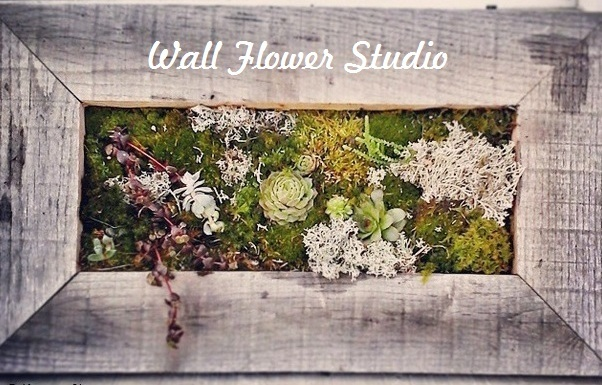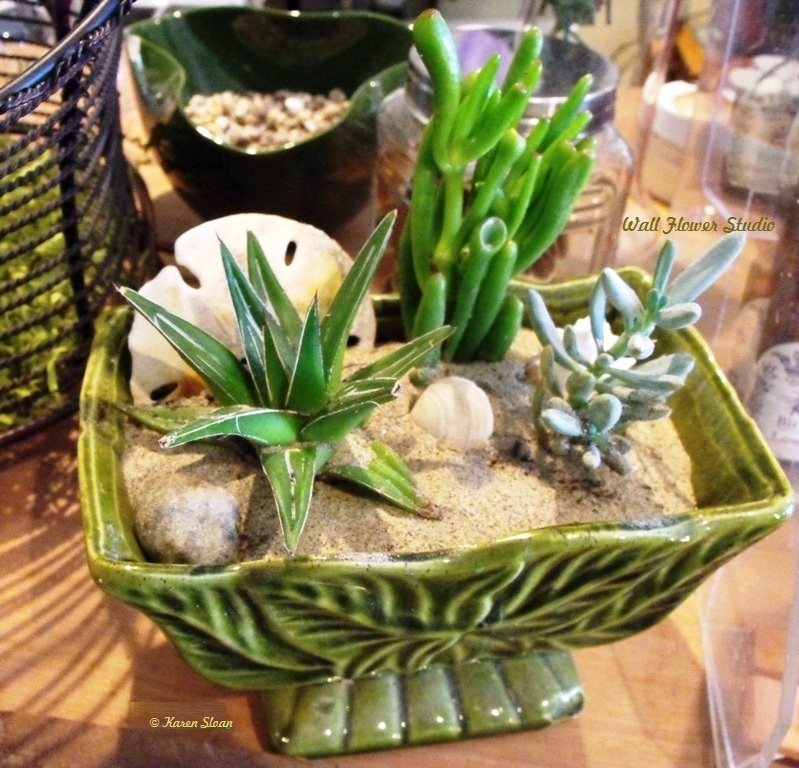table div table+table div table,table.module-1{width:3.77%;float:left;padding:0}table div table+table div table a{border:0 none;text-decoration:none}table div table+table div table img{width:100%!important;border:0 none;text-decoration:none}table div table+table div table td{width:100%;padding:0 20px 20px 0}/* styles */ Hello Friends, The leaves have fallen and our plants have been brought inside. Now is the time to consider how our houseplants can become a focal point in our design and décor. Not just for their beauty, but for the many health benefits they offer! Plants clean the air inside our homes and bring a welcome sense of calm to our living space. A few suggestions: Our dry winter houses are the opposite of what humidity loving tropical plants prefer, so consider hanging a tropical plant in your bathroom & let the humidity from the shower help it grow. This goes for air plants, too! Having said that, succulents love the dry, warm air. However, they may need topping up with more frequent drinks as the soil can dry out quicker. (True cacti on the other hand should not be watered from November to March. If you're unsure which plant is which, send me a photo and I will help with any watering questions you may have.) Place multiple plants in a corner or grouped on an end table to create a greenhouse-like space. Put a plant next to your bed for a natural air filter. Greenery can be up on pedestals and stands to enhance their beauty. As we reconnect with nature indoors, we can cultivate a relaxed and welcoming environment by adding just a few living plants to green up our lives. Explore and enjoy!table div table+table+table+table div table{width:100%;padding:0}table div table+table+table+table div table img{width:96.23%;padding:0;float:none}table div table+table+table+table div table td{width:100%;padding:0 1.88% 18px}/* styles */## Upcoming workshops

 table div table+table+table+table+table+table div table,table.module-5{width:3.77%;float:right;padding:0}table div table+table+table+table+table+table div table a{border:0 none;text-decoration:none}table div table+table+table+table+table+table div table img{width:100%!important;border:0 none;text-decoration:none}table div table+table+table+table+table+table div table td{width:100%;padding:0 0 20px 20px}/* styles */ Pocket vertical succulent garden These airy mesh pouches stuffed with natural moss & succulents create charming vertical gardens. They're excellent for smaller spaces where the only way to grow is up. Join me at the store and I'll show you how to create these lovely plant pockets to take home, nurture & display. Alternatively, offer it as a hostess gift, or give it to a friend over the holidays! Date: Sat. Nov. 25th, 10-noon. Fee: \$30 To register contact Karen at: 705.286.6999 - sloanartgallery@gmail.com or message her on Facebook - Thank you!
 table div table+table+table+table+table+table+table div table{width:100%;padding:0}table div table+table+table+table+table+table+table div table img{width:96.23%;padding:0;float:none}table div table+table+table+table+table+table+table div table td{width:100%;padding:0 1.88% 18px}/* styles */## Exciting November happenings in Minden

 table div table+table+table+table+table+table+table+table+table div table,table.module-8{width:3.77%;float:right;padding:0}table div table+table+table+table+table+table+table+table+table div table a{border:0 none;text-decoration:none}table div table+table+table+table+table+table+table+table+table div table img{width:100%!important;border:0 none;text-decoration:none}table div table+table+table+table+table+table+table+table+table div table td{width:100%;padding:0 0 20px 20px}/* styles */ Festival of Trees Wall Flower Studio is happy to create and donate a living succulent wreath for the event. - Purchase raffle tickets and I'll cross my fingers that you win! The winner would be welcome to contact me for care/maintenance & instructions.
 table div table+table+table+table+table+table+table+table+table+table div table,table.module-9{width:3.77%;float:left;padding:0}table div table+table+table+table+table+table+table+table+table+table div table a{border:0 none;text-decoration:none}table div table+table+table+table+table+table+table+table+table+table div table img{width:100%!important;border:0 none;text-decoration:none}table div table+table+table+table+table+table+table+table+table+table div table td{width:100%;padding:0 20px 20px 0}/* styles */ Lights & Delights Friday Nov. 17th 6-8 pm Fun for all ages! The perfect way to begin the holiday season.
 table div table+table+table+table+table+table+table+table+table+table+table div table,table.module-10{width:3.77%;float:right;padding:0}table div table+table+table+table+table+table+table+table+table+table+table div table a{border:0 none;text-decoration:none}table div table+table+table+table+table+table+table+table+table+table+table div table img{width:100%!important;border:0 none;text-decoration:none}table div table+table+table+table+table+table+table+table+table+table+table div table td{width:100%;padding:0 0 20px 20px}/* styles */ Santa's coming! November 25th 11:30 am. Come see him in person!
 table div table+table+table+table+table+table+table+table+table+table+table+table div table{width:100%;padding:0}table div table+table+table+table+table+table+table+table+table+table+table+table div table img{width:96.23%;padding:0;float:none}table div table+table+table+table+table+table+table+table+table+table+table+table div table td{width:100%;padding:0 1.88% 18px}/* styles */table div table+table+table+table+table+table+table+table+table+table+table+table+table div table,table.module-12{width:3.77%;float:left;padding:0}table div table+table+table+table+table+table+table+table+table+table+table+table+table div table a{border:0 none;text-decoration:none}table div table+table+table+table+table+table+table+table+table+table+table+table+table div table img{width:100%!important;border:0 none;text-decoration:none}table div table+table+table+table+table+table+table+table+table+table+table+table+table div table td{width:100%;padding:0 20px 20px 0}/* styles */ November's Flower - Chrysanthemum As the birth flower for November, the chrysanthemum is one of the most colourful flowers that blooms in fall; a season that's known for few (if any) blooms in our neck of the woods! Therefore, the chrysanthemum symbolizes joy and beauty despite the oncoming colder months.
 table div table+table+table+table+table+table+table+table+table+table+table+table+table+table div table{width:100%;padding:0}table div table+table+table+table+table+table+table+table+table+table+table+table+table+table div table img{width:96.23%;padding:0;float:none}table div table+table+table+table+table+table+table+table+table+table+table+table+table+table div table td{width:100%;padding:0 1.88% 18px}/* styles */# November Poem

“November comes
And November goes,
With the last red berries
And the first white snows.

With night coming early,
And dawn coming late,
And ice in the bucket
And frost by the gate.

The fires burn
And the kettles sing,
And earth sinks to rest
Until next spring.”
― Clyde Watson

 table div table+table+table+table+table+table+table+table+table+table+table+table+table+table+table+table div table{width:100%;padding:0}table div table+table+table+table+table+table+table+table+table+table+table+table+table+table+table+table div table img{width:96.23%;padding:0;float:none}table div table+table+table+table+table+table+table+table+table+table+table+table+table+table+table+table div table td{width:100%;padding:0 1.88% 18px}/* styles */table div table+table+table+table+table+table+table+table+table+table+table+table+table+table+table+table+table div table,table.module-16{width:3.77%;float:left;padding:0}table div table+table+table+table+table+table+table+table+table+table+table+table+table+table+table+table+table div table a{border:0 none;text-decoration:none}table div table+table+table+table+table+table+table+table+table+table+table+table+table+table+table+table+table div table img{width:100%!important;border:0 none;text-decoration:none}table div table+table+table+table+table+table+table+table+table+table+table+table+table+table+table+table+table div table td{width:100%;padding:0 20px 20px 0}/* styles */ Following a tradition inaugurated by King George V in 1919, November 11th is the day most countries recall the end of hostilities of World War I. Hostilities formally ended at the 11th hour of the 11th day of the 11th month. This is the time of year we are filled with gratitude and remember the many who sacrificed their lives for the betterment of our own.
 table div table+table+table+table+table+table+table+table+table+table+table+table+table+table+table+table+table+table div table{width:100%;padding:0}table div table+table+table+table+table+table+table+table+table+table+table+table+table+table+table+table+table+table div table img{width:96.23%;padding:0;float:none}table div table+table+table+table+table+table+table+table+table+table+table+table+table+table+table+table+table+table div table td{width:100%;padding:0 1.88% 18px}/* styles */In the spirit of gratitude, thank you for receiving my newsletter, and participating in the journey of my wee shop!

 table div table+table+table+table+table+table+table+table+table+table+table+table+table+table+table+table+table+table+table+table div table{width:100%;padding:0}table div table+table+table+table+table+table+table+table+table+table+table+table+table+table+table+table+table+table+table+table div table img{width:96.23%;padding:0;float:none}table div table+table+table+table+table+table+table+table+table+table+table+table+table+table+table+table+table+table+table+table div table td{width:100%;padding:0 1.88% 18px}/* styles */Look for another newsletter in early December.
Have a lovely month!
~ Karen

 table div table+table+table+table+table+table+table+table+table+table+table+table+table+table+table+table+table+table+table+table+table+table div table{width:100%;padding:0}table div table+table+table+table+table+table+table+table+table+table+table+table+table+table+table+table+table+table+table+table+table+table div table img{width:96.23%;padding:0;float:none}table div table+table+table+table+table+table+table+table+table+table+table+table+table+table+table+table+table+table+table+table+table+table div table td{width:100%;padding:0 1.88% 18px}/* styles */table.module-23{width:0%;padding:0}table div table+table+table+table+table+table+table+table+table+table+table+table+table+table+table+table+table+table+table+table+table+table+table+table div table{width:0%;float:none;margin-left:auto;margin-right:auto;padding:0}table div table+table+table+table+table+table+table+table+table+table+table+table+table+table+table+table+table+table+table+table+table+table+table+table div table a{border:0 none;text-decoration:none}table div table+table+table+table+table+table+table+table+table+table+table+table+table+table+table+table+table+table+table+table+table+table+table+table div table img{width:100%!important;border:0 none;text-decoration:none}table div table+table+table+table+table+table+table+table+table+table+table+table+table+table+table+table+table+table+table+table+table+table+table+table div table td{width:100%;padding:0}/* styles */
 Like   Tweet   Pin   in